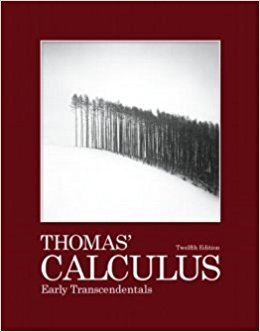×
×

# Solutions for Chapter 10: Infinite Sequences and Series## Full solutions for Thomas' Calculus Early Transcendentals | 12th Edition

ISBN: 9780321588760Solutions for Chapter 10: Infinite Sequences and Series

Solutions for Chapter 10
4 5 0 373 Reviews
27
1
##### ISBN: 9780321588760

Since 92 problems in chapter 10: Infinite Sequences and Series have been answered, more than 8016 students have viewed full step-by-step solutions from this chapter. This expansive textbook survival guide covers the following chapters and their solutions. Thomas' Calculus Early Transcendentals was written by and is associated to the ISBN: 9780321588760. This textbook survival guide was created for the textbook: Thomas' Calculus Early Transcendentals, edition: 12. Chapter 10: Infinite Sequences and Series includes 92 full step-by-step solutions.

Key Calculus Terms and definitions covered in this textbook
• Anchor

See Mathematical induction.

• Annual percentage yield (APY)

The rate that would give the same return if interest were computed just once a year

• Cone

See Right circular cone.

• Convergence of a sequence

A sequence {an} converges to a if limn: q an = a

• Cycloid

The graph of the parametric equations

• Directed distance

See Polar coordinates.

• Extracting square roots

A method for solving equations in the form x 2 = k.

• Heron’s formula

The area of ¢ABC with semiperimeter s is given by 2s1s - a21s - b21s - c2.

• Identity

An equation that is always true throughout its domain.

• Midpoint (on a number line)

For the line segment with endpoints a and b, a + b2

• One-to-one rule of logarithms

x = y if and only if logb x = logb y.

• Placebo

In an experimental study, an inactive treatment that is equivalent to the active treatment in every respect except for the factor about which an inference is to be made. Subjects in a blind experiment do not know if they have been given the active treatment or the placebo.

• Power regression

A procedure for fitting a curve y = a . x b to a set of data.

• Probability of an event in a finite sample space of equally likely outcomes

The number of outcomes in the event divided by the number of outcomes in the sample space.

• Root of an equation

A solution.

• RRAM

A Riemann sum approximation of the area under a curve ƒ(x) from x = a to x = b using x1 as the right-hand end point of each subinterval.

• Vertex of an angle

See Angle.

• Vertices of a hyperbola

The points where a hyperbola intersects the line containing its foci.

• Weighted mean

A mean calculated in such a way that some elements of the data set have higher weights (that is, are counted more strongly in determining the mean) than others.

• Ymin

The y-value of the bottom of the viewing window.

×